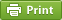Werner's Blog — Opinion, Analysis, Commentary
The Dorfman-Steiner Theorem and increasing returns to scale

How much advertisement is sufficient? This is a classical question to which the answer has been known for more than sixty years. The Dorfman-Steiner Theorem in economics can be derived quite simply by taking a profit function where quantity sold $$q$$ depends on price $$p$$ and advertisment $$a$$, and goods sold are produced at constant marginal cost $$c$$. Then $\pi=(p-c)\cdot q(p,a)-a$ and the corresponding two first-order conditions are $\frac{\mathrm{d}\pi}{\mathrm{d} p} =q(p,a)+(p-c)\frac{\partial q}{\partial p}=0$ and $\frac{\mathrm{d}\pi}{\mathrm{d} a} =(p-c)\frac{\partial q}{\partial a}-1=0$ Introduce the two elasticities $\eta\equiv-\left(\frac{\partial q}{\partial p}\right)\left(\frac{p}{q}\right) >0\quad\mathrm{and}\quad \theta\equiv\left(\frac{\partial q}{\partial a}\right)\left(\frac{a}{q}\right)>0$ which allows us to rewrite the two first-order conditions as $\eta=\frac{p}{p-c} \quad\mathrm{and}\quad \frac{a}{p\cdot q}=\frac{p-c}{p}\theta$ Combining the two conditions yields $\frac{a}{p\cdot q}= \frac{\theta}{\eta}$ which says that the optimal level of advertisement (as a share of revenue) is equal to the ratio of advertising elasticity and price elasticity. Put another way, advertisment increases with its effectiveness but decreases with price elasticity. Advertisement expenditures tend to be larger in price-inelastic markets.

This simple rule has been extended to a dynamic framework as well. Whereas the Dorfman-Steiner theorem only holds for a single period, the problem becomes more complicated when the effect of advertisement is slow and lags behind. In a multi-period world, Levy an Simon (1989) find that the optimizing decision rule also depends on the retention rate of customers and the discount rate for the cost of capital.

Another important question is what happens to the Dorfman-Steiner condition in the presence of increasing returns to scale. Let us extend the simple model by allowing the unit cost to depend on output so that the output elasticity of unit cost is given by $\zeta\equiv-\frac{c(q)}{q}\frac{q}{c(q)}>0$ This elasticity is defined as a positive number for increasing returns to scale, which means that an increase in output lowers the per-unit cost of production. Then the profit function becomes $\pi=[p-c(q)]\cdot q(p,a)-a$ and is maximized with respect to output $$q$$ and advertisement $$a$$. The mark-up is now not only a function of the price elasticity $$\eta$$ but also the increasing returns factor $$\zeta$$. The stronger the increasing returns to scale, the lower the markup. The original Dorfman-Steiner condition was expressed with respect to revenue $$p\cdot q$$. As we are considering increasing returns to scale, it makes more sense to express the Dorfman-Steiner condition with respect to production cost $$c\cdot q$$. Then $\frac{a}{c\cdot q}=\theta\cdot\frac{1-\zeta}{\eta-1}$ So the increasing returns to scale version of Dorfman-Steiner says that the advertisement expenditures to production cost increases with the advertisement effectiveness (the elasticity $$\theta$$) and decreases with the price elasticity $$\eta$$, but is also dampened by the effect of increasing returns to scale (the unit cost elasticity $$\zeta$$). In other words, advertisement should be very small when increasing returns to scale are large. Perhaps that explains why Boeing and Airbus do not need to advertise much. Note that the new version of the Dorfman-Steiner condition merely extends the original condition but does not substantively change it. Increasing returns to scale affects the mark-up, but not the share of advertisement expenditure relative to revenue.

Posted on Thursday, March 31, 2016 at 17:45 — #EconomicsRecent Blog Entries

Topics

Months

Subscribe to RSS feed© 2023  Prof. Werner Antweiler, University of British Columbia.